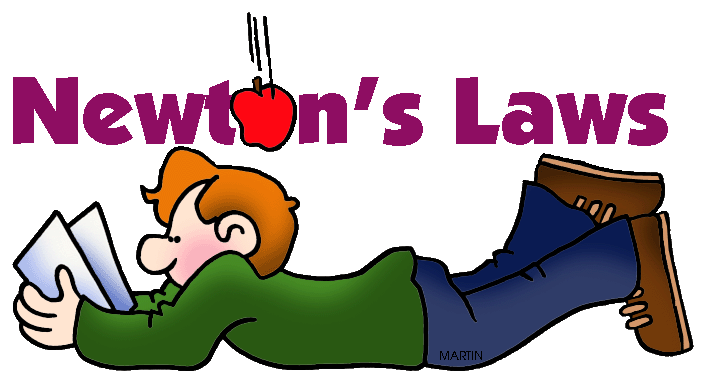Newton's Laws of Motion
4 years ago
cfloydsm
Save
Edit
Host a game
Live GameLive
Homework
Solo Practice
Practice20 QuestionsShow answers
• Question 1
30 seconds
Q. An object at rest will _______ if no outside forces are applied.
Stay at rest
Increase Velocity
Decrease Mass
• Question 2
30 seconds
Q. Momentum is related to Newton's first law.
True
False
• Question 3
30 seconds
Q. A greater applied force is required to move an object with a greater mass than one with a smaller mass.
True
False
• Question 4
30 seconds
Q. The SI units for force are...
Kilograms
Newtons
Meters per second
Pounds
• Question 5
60 seconds
Q. If a baseball and a cannonball are dropped from the same height at the same time, which ball will hit the ground first?
the cannonball
the baseball
The balls land at the same time.
the ball with the larger volume
• Question 6
60 seconds
Q. If an action force is a cue ball (white) hitting a billiard ball (various colors) when playing pool, then the reaction force is
exerted on the table
exerted on all the other billiard balls.
not present
exerted by the billiard ball on the cue ball.
• Question 7
60 seconds
Q. A crumpled piece of paper hits the ground before a flat sheet of paper because
the acceleration of gravity is greater on the  crumpled paper.
there is more air resistance against the flat paper.
the crumpled paper is more massive
the crumpled paper is less massive.
• Question 8
60 seconds
Q. According to Newton’s first law of motion, a moving object that is not acted on by an unbalanced force will
remain in motion.
eventually come to a stop.
change its momentum.
accelerate.
• Question 9
60 seconds
Q. An ice skater at rest pushes against a sled at rest, causing both the skater and sled to move away from each other with different accelerations. This is an example best described by
Newton’s first law of motion for objects at rest.
Newton’s first law of motion for objects in motion.
Newton’s second law of motion.
Newton’s third law of motion.
• Question 10
60 seconds
Q. A sled sliding on a flat, icy surface with a constant velocity is best described by
Newton’s first law of motion for objects at rest.
Newton’s first law of motion for objects in motion.
Newton’s second law of motion.
Newton’s third law of motion.
• Question 11
60 seconds
Q. Which of the following explains the difference between speed and velocity?
One has motion, and the other does not.
One has direction, and the other does not.
One involves time, and the other does not.
One involves acceleration, and the other does not.
• Question 12
60 seconds
Q. The gravitational pull is greater between two objects that
have greater masses.
have rougher surfaces.
are farther apart.
are moving at greater speed
• Question 13
30 seconds
Q. Dividing the total distance traveled by the total time is how to calculate
average speed.
average velocity.
average acceleration.
• Question 14
60 seconds
Q. A hiker’s velocity begins at 1.8 m/s uphill and changes to 1.5 m/s uphill. How do you know that the hiker has a negative acceleration?
His direction changed.
His direction was unchanged.
His speed increased.
His speed decreased.
• Question 15
60 seconds
Q. How does Newton’s third law explain how a rocket takes off?
The rocket’s acceleration is positive, while the gasses acceleration is negative but the direction is the same.
The rocket is at rest until ignition.
The hot gasses move in one direction, while the rocket moves in the opposite direction but with equal force.
• Question 16
30 seconds
Q. Acceleration is caused by a force acting on a mass.
Newton’s first law of motion
Newton’s second law of motion
Newton’s third law of motion
• Question 17
30 seconds
Q. For a force on an object there is an equal and opposite force.
Newton’s first law of motion
Newton’s second law of motion
Newton’s third law of motion
• Question 18
30 seconds
Q. Without any force to change it, an object at rest stays at rest and an object in motion stays in motion
Newton’s first law of motion
Newton’s second law of motion
Newton’s third law of motion
• Question 19
60 seconds
Q. If a force of 26 N is exerted on two balls, one with a mass of 0.52 kg and the other with a mass of 0.78 kg, which ball will have the greater acceleration?  (F=ma)